### A Powerful and Secondary Review: ASIC Design concepts

In the previous post on MOSFETs, I introduced how PMOS and NMOS Structure and Characteristic functionality works. This post is based on the MOSFET prerequisites, which introduces some of the important ASIC design concepts as listed below.
• Small Signal Model
• Parasitic Capacitance and fT

Here, I introduce the concept of Small Signal. Firstly, before we do this analysis, we need to bias the transistor.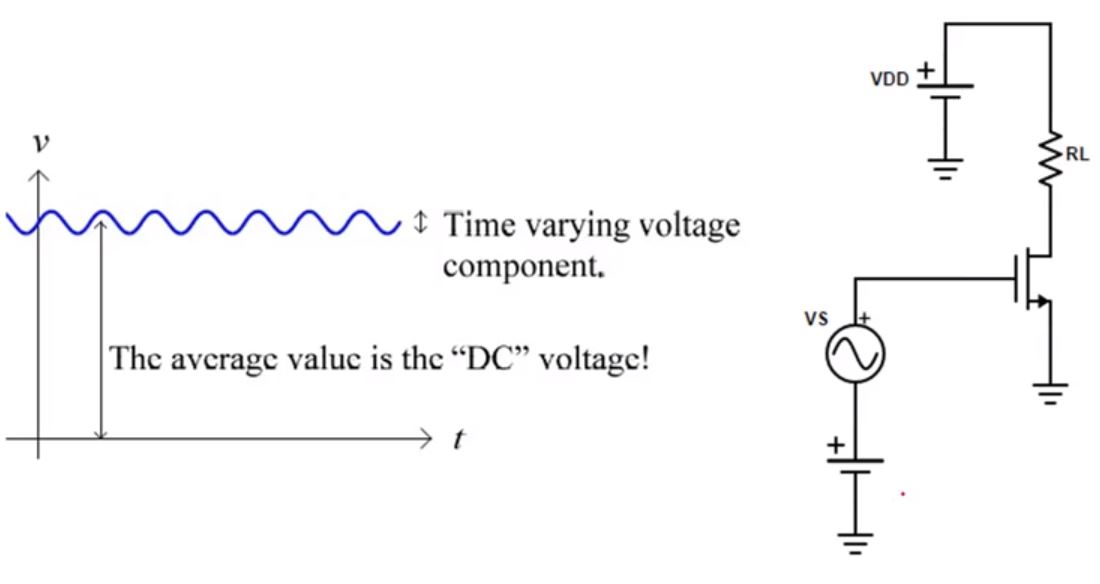FIG 1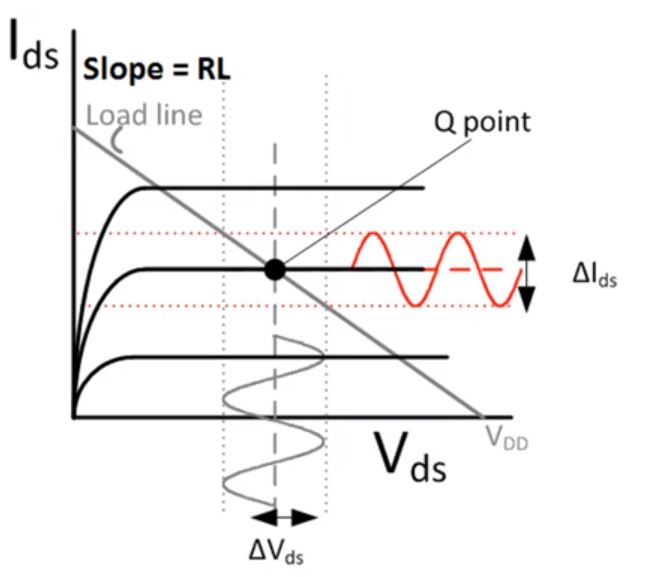FIG 2

Biasing means we need to set the work-point/operation-point of the transistor. So, 'Vs' in FIG 1 is our small signal, which means that the amplitude is low, say 1mV. Since we have a DC (FIG 1) along with AC as well, so the Gate Voltage has a DC and AC value at the same time.

Let us, for a while, forget about this Small Signal and let us bias the transistor by applying a bias or positive voltage to the Gate. In the above circuit, the load RL is connected to the source voltage VDD.
Now, if you look at FIG 2, we have chosen an operating point (Q point). To choose this point, we choose VGS. Also, by changing the transistor size (W/L), we are gating the current (IDS) from the transistor.

Let us say, the long channel is high, and the lambda value is 0. Assuming (from the lower VGS to higher VGS value) that the constant current curves have value:- VGS1, VGS2, VGS3. Thus, we are setting VGS2 as the VGS. Now we can set-up the DC point for the current value (ID2). With all this, we can get the VDS by using this: VDS = VDD - RL.ID. Thus, the VDS value is on the X-axis (marking on the X-axis), and we can assume it as VD2. The intersection of ID2 and VD2 is the bias point, and here, the transistor is working in the saturation region. In this region, VDS > VGS - VT and, VGS2 > VT which turns-on the transistor.

Now, let us consider the AC signal and assuming the time-varying voltage component with its amplitude as 1mV. Thus total VG = VGS2 + VS = 1V + 1mV cos(wt). Refer FIG 1. We have the alternating signal for the Gate, Drain, and Current ID on RL.

To show this alternating small signal on the graph (FIG 2), we will now consider the top and bottom limit of AC voltage signal which would be [1V + 1mV] and [1V - 1mV] respectively. Similarly, we are going to have fluctuation in the current values below and above ID2 which can be named as ID1 and ID3. We can also mark the above and below of  the chosen DC voltage value VD2 for the AC voltage as VD1 and VD3. The current and voltage values are equal to ID3 - ID2. and VDS3 - VDS2

So as per the small-signal analysis, we can see if we apply the AC Voltage and DC Voltage to the Gate of the transistor, we will be able to get the varying current and drain voltage and they are all small signal.

Small Signal Model (Analysis):• gm = (δ Ids) / (δ Vgs) : Fluctuation of current over voltage of gate-source.
• gm = √ 2 . μn .Cox (W/L) . IDS ]
• gm = 2ID / (VGS - VT)gm = [( Ids3 - Ids1) / ( Vgs3 - Vgs3 )]

Transconductance (gm) is used as a parameter that depends on certain transistor parameters and it is constant (assumption). Also, VOverdrive = VGS - VT. The overdrive voltage is mostly positive for the transistor which is ON. gm increases as ID increases and it depends on transistor size and DC bias.The above figure shows the small-signal model of the transistor. Here, the output resistance is ro (it is the fluctuation of Drain-Source Voltage to Current) and current source is gmVgs. Once we find the value of gm, we can find the current value between D and S. Thus, on applying a wave to Gate (after VS), we can notice the Drain current (ID) and VD getting fluctuation. The fluctuation of VD/ID = ro.

• ro = (δVds / δIds) = VA / ID : here, ID is the bias current.
• Gain = VO / VS = gm. (RL||ro)
Also, since VA is high for Long-Channel transistors (L), therefore ro is high for Long-channel transistors as well. But, in recent technologies, we are observing lower values of L and ro (short-channel device).

Let us say, we have our model below. When we are doing the AC analysis, we have to first make the DC part zero, and it works for the DC as well. So, when we are trying to find our DC point, and if we find any AC source then it is set to zero. But, while finding the AC values, all the DC voltage is set to zero. Here, only AC Gain is being considered and the DC value is just to set the operation point.Transistor working in detail: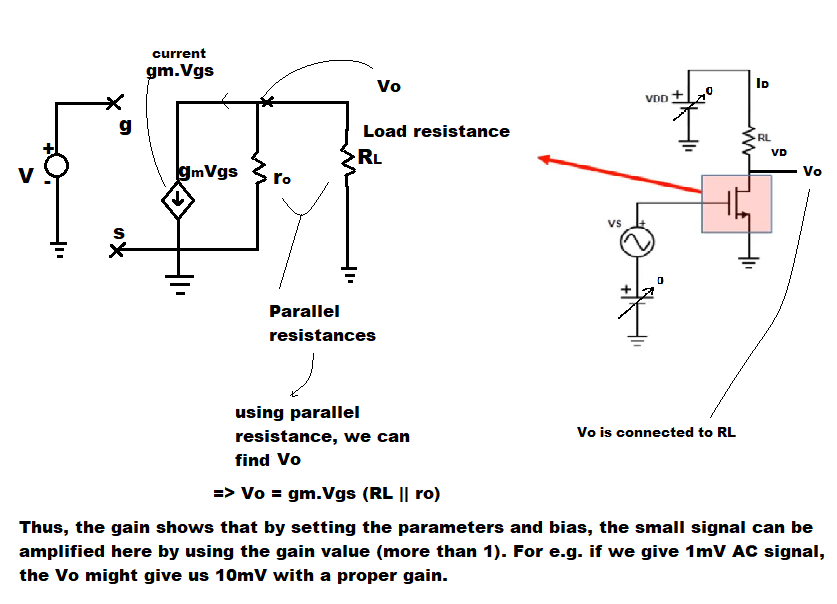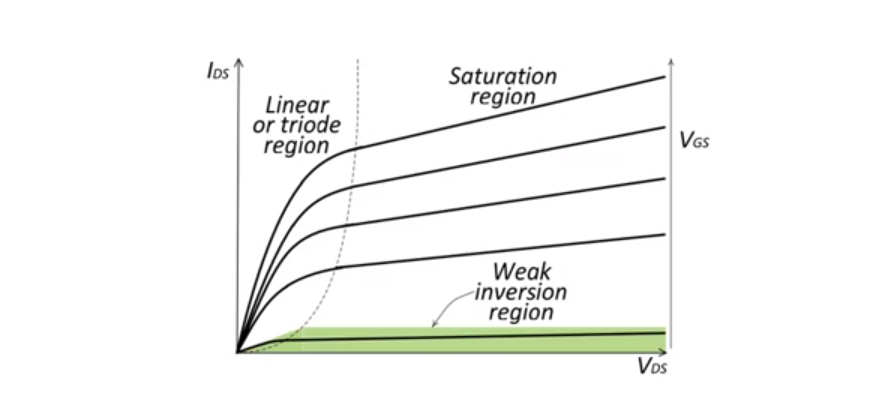Also, we always try to work in the saturation region and try to get a higher output resistance. In the above figure, the slope of IDS and VDS is low in the saturation region (slope = IDS / VDS => which is inverse of ro). Hence, when the slope is high the value of ro is low and vice versa. Therefore, in the saturation region the output resistance of the transistor is high even if it is a long channel. In the linear or triode region, the slope is high and hence the output resistance is low, thus we avoid making the transistor work in triode region.

Parasitic Capacitances: These are unwanted capacitances in the circuit, but still are part of the transistor. Together with the resistances in the circuit, they put an upper limit to the speed of the transistor.We cannot avoid parasitic capacitances. While working the lower frequency it doesn't really matter. But while working with higher frequencies, especially RF circuits as they work on a high frequency, we always have to take parasitic capacitances because, as the impedance of the capacitance = 1/(jcω) and for low frequency we can say that ω value is, and it can be said that CGD (Gate-Drain Capacitance) is open. So, as we increase the frequency, the CGD acts as an impedance which changes the behavior of the transistor.

=> Parasitics limit the transistor.

Where are these parasitics coming from?

There is this depletion region between C1 and C2 => as they are N-type and P-type semiconductors respectively. The capacitance is from Source to Bulk, or CDB i.e. from Drain to Bulk, have a depletion region. There is a very small region where Gate overlaps the Source, and here the capacitance is called Overlapping Capacitance or Gate to Source Capacitance. Capacitance C3 is from the Channel to Bulk. Capacitance C6 is from Gate to Bulk (with an oxide in between). The capacitance can be in between the channel and the gate and the channel and bulk as well, hence we are considering here both C3 and C6. The other one can be C5 between Gate and Drain.

So, we consider three regions here, and most of the time we prefer the transistor to work in the saturation region. In Saturation region, the Gate-Source Capacitance (CGS) can be calculated as 2/3(W.L.Cox) + W.Cov. Here, Cox = Oxide Capacitance and Cov = Overlapping Capacitance. But when the transistor goes into the Triode region, it will be same as CGD  which is the half of W.L.Cox. Generally, we work in the Saturation region so we know that the highest capacitance in this circuit is CGD.

So, if we observe the transistor, the input capacitance CIN is equal to CGS. Here we can see that it is even higher than the CGD  which is in the Saturation region. Thus, we need to take extra care while considering CGS.

High-Frequency Small-Signal model:When we get into a really High-Frequency, the model gets a bit complicated. Here, gmb is related to the Bulk, but it is smaller than gm (gmb < gm), and sometimes it can be ignored, but we can consider this if we are counting smaller values as well.

To measure the speed of a Transistor, we use unity-gain frequency fT. It is the frequency at which the current that enters the gate iin equals the current that flows through the channel iout.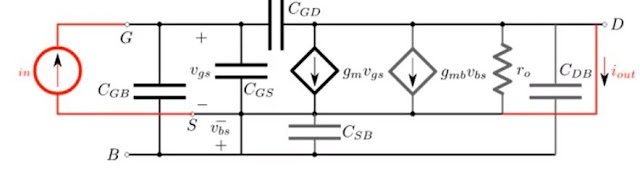=> When, (iout / is ) = 1

Hence, fT is the frequency at which the current gain equals 1 which means that it is the maximum speed that we can get from this transistor. Here, we can see that the fT depends on gm and CGS. High gm means that we have a fast transistor. So, as the current increases => gm increases (as per their relationship equation), considering here that power consumption is not an issue as the power would increase with the increase in current values.

Here, we also consider the CGS tradeoff. So as we decrease the CGS, which depends on WL. Therefore we can make the transistor smaller. The interesting thing here to notice is that when we want to increase the Power or Current to make the transistor work faster, we will need to increase its size as well to avoid it to work in weak inversion, it won't work in the saturation region. Thus, this is a tradeoff between the Transistor size and capacitance with its speed and power. We don't want to burn our transistor by applying higher power, isn't it?! So, be careful about this, that is all I want to say.We really need to increase the power if we want a fast transistor here. I am not sure right now what Apple Inc. recently (2019-2020) did by experimenting with its iPhone battery system for the 5G RF network ICs as this new technology consumes a lot of power.

In the above figure, iout / is affects the frequency and it drops down to provide the current gain = 1 (as we know DC gain, gm, with RL || ro ). So, by increasing the frequency, the gain will decrease to a point at which it becomes equal to 1.

{\displaystyle \infty }

1.